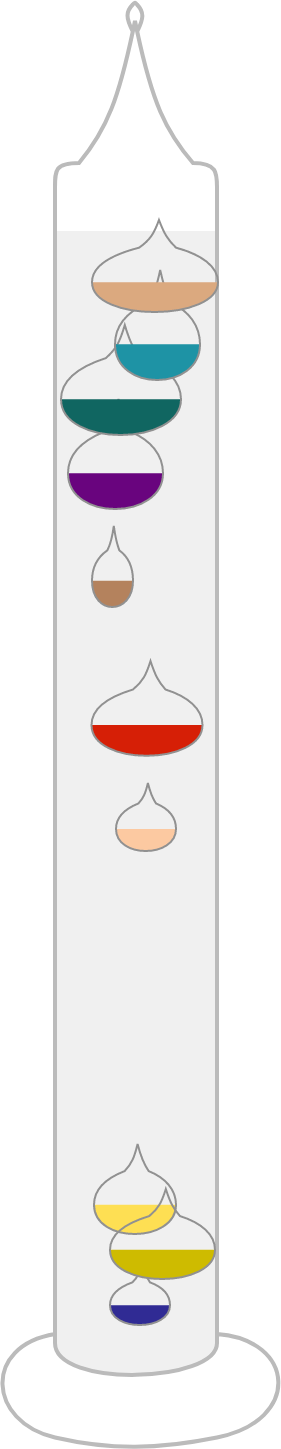# Galileo Thermometer!The glass bulbs of a Galileo thermometer are sealed such that their densities are constant. The clear liquid in which the bulbs are submerged is ethanol, which changes density depending on the surrounding temperature.

Each bulb is labeled with the temperature at which its density is equal to that of the ethanol. For example, if the ethanol temperature is $20^\circ \text{C},$ then the bulb labeled $20^\circ \text{C}$ would have the same density as the ethanol.

At $25^\circ\text{C}$ ethanol temperature, what do you observe inside the thermometer?

Details and Assumptions:

• The density of ethanol decreases with increasing temperature.
×